Question

# [1 -1 0 0 -2 0] 1 4 -4 0 0 -8 0 (1 point) Let...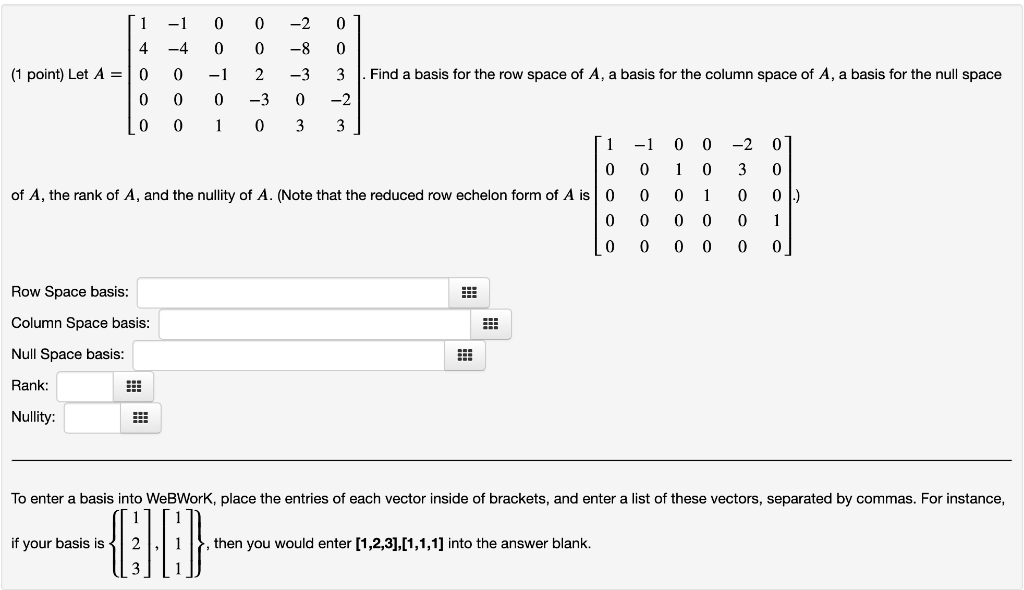[1 -1 0 0 -2 0] 1 4 -4 0 0 -8 0 (1 point) Let A = 10 0 -1 2 -3 3 . Find a basis for the row space of A, a basis for the column space of A, a basis for the null space 0 0 0 -3 0 -2 Lo 0 1 0 3 3] [1 -1 0 0 -2 01 0 0 1 0 3 0 of A, the rank of A, and the nullity of A. (Note that the reduced row echelon form of A is 0 0 0 1 0 0 .) 0 0 0 0 0 1 Lo 0 0 0 0 0 Row Space basis: Column Space basis: Null Space basis: Rank: Nullity: To enter a basis into WebWork, place the entries of each vector inside of brackets, and enter a list of these vectors, separated by commas. For instance, if your basis is | 2,1l}, then you would enter [1,2,3],[1,1,1) into the answer blank. 311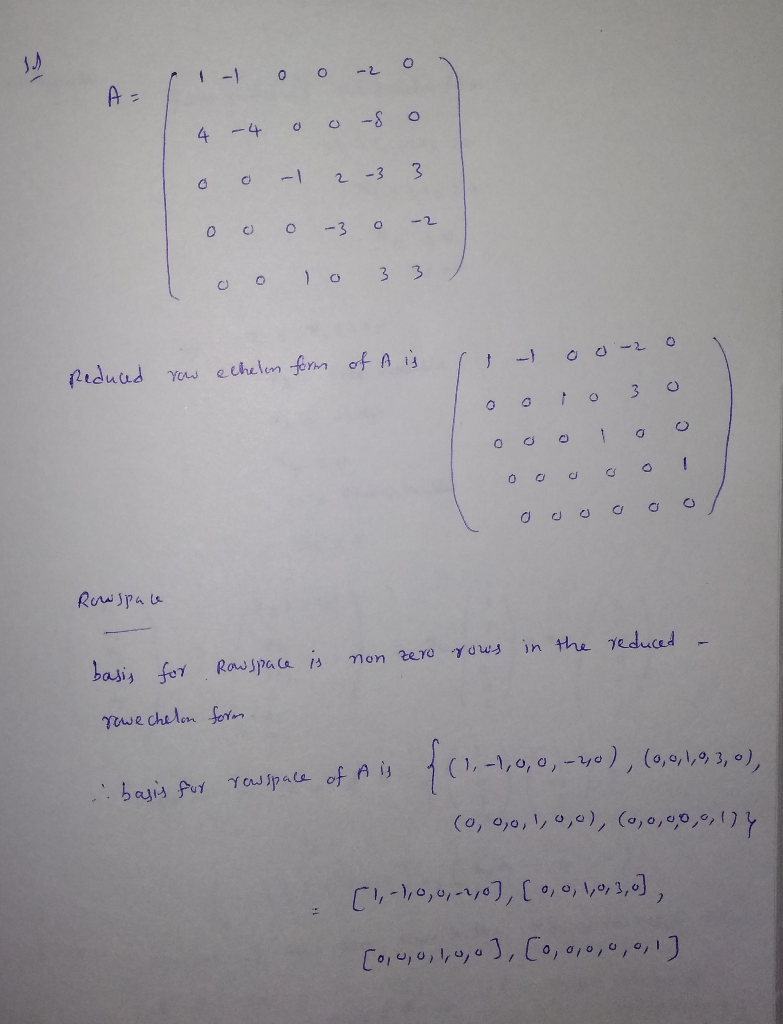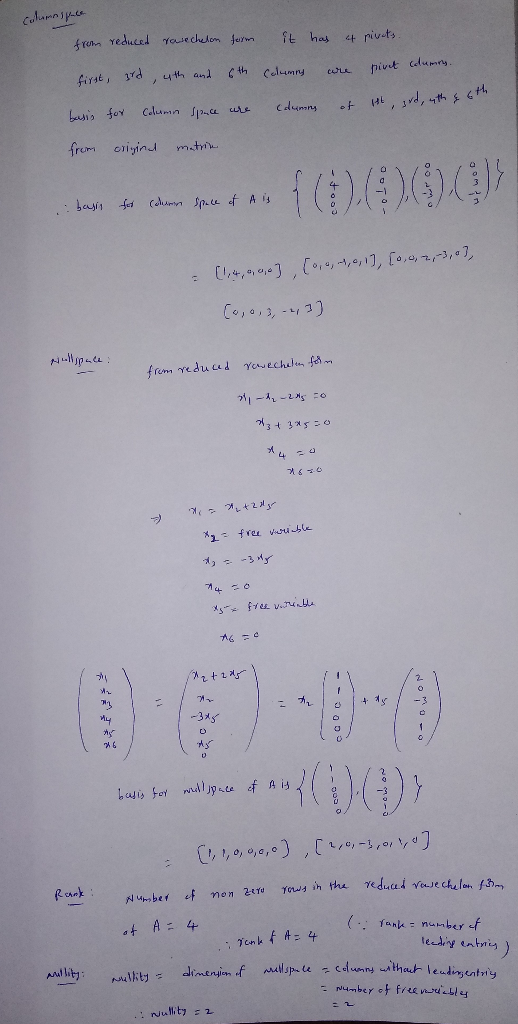#### Earn Coins

Coins can be redeemed for fabulous gifts.

Similar Homework Help Questions
• ### (1 point) Find an orthonormal basis of the plane X1 + 4x2 – x3 = 0....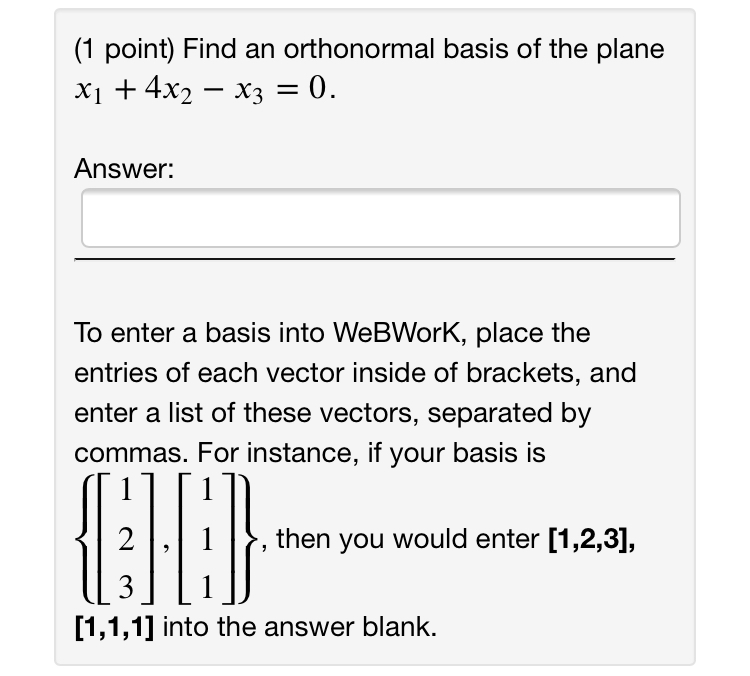(1 point) Find an orthonormal basis of the plane X1 + 4x2 – x3 = 0. Answer: To enter a basis into WebWork, place the entries of each vector inside of brackets, and enter a list of these vectors, separated by commas. For instance, if your basis is 2 then you would enter [1,2,3], 3 [1,1,1) into the answer blank.

• ### (1 point) Let A = 2 2 | -4 1-2 6 -3 -3 3 3 -3...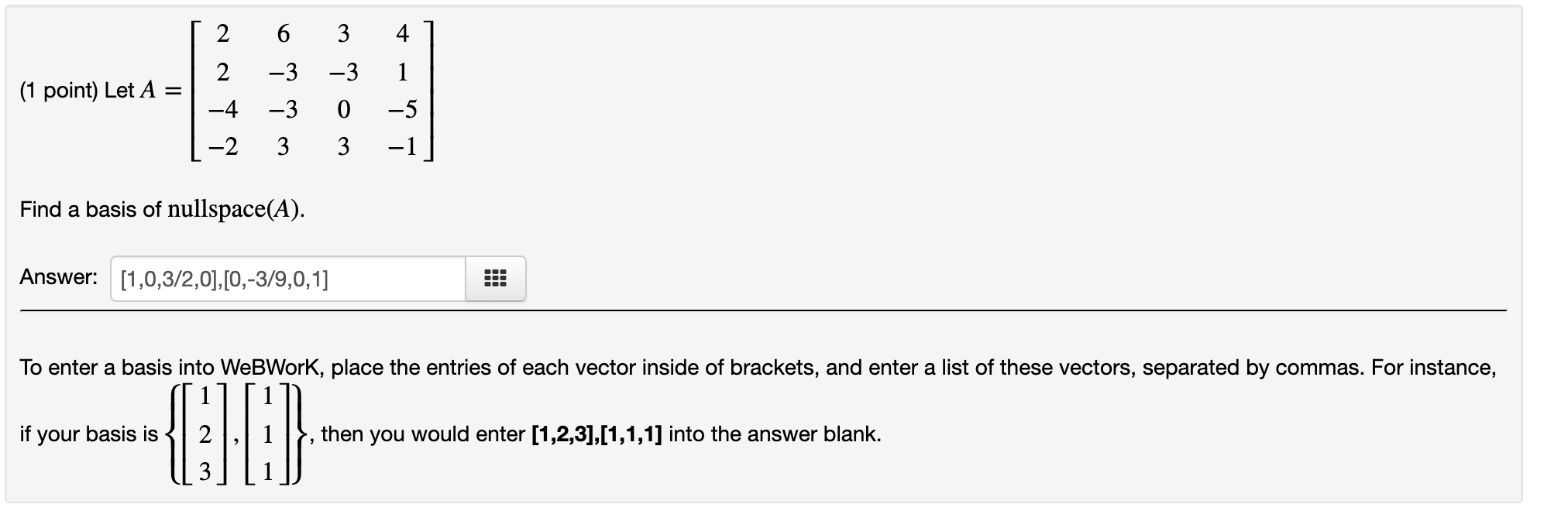(1 point) Let A = 2 2 | -4 1-2 6 -3 -3 3 3 -3 0 3 4 7 1 -5 -1 Find a basis of nullspace(A). Answer: [1,0,3/2,0], [0,-3/9,0,1] To enter a basis into WeBWork, place the entries of each vector inside of brackets, and enter a list of these vectors, separated by commas. For instance, if your basis is 12, 1 }, then you would enter [1,2,3],[1,1,1) into the answer blank. U 3 ||1

• ### T0 0 0 ] (1 point) The matrix A = -5 5 10 has two real...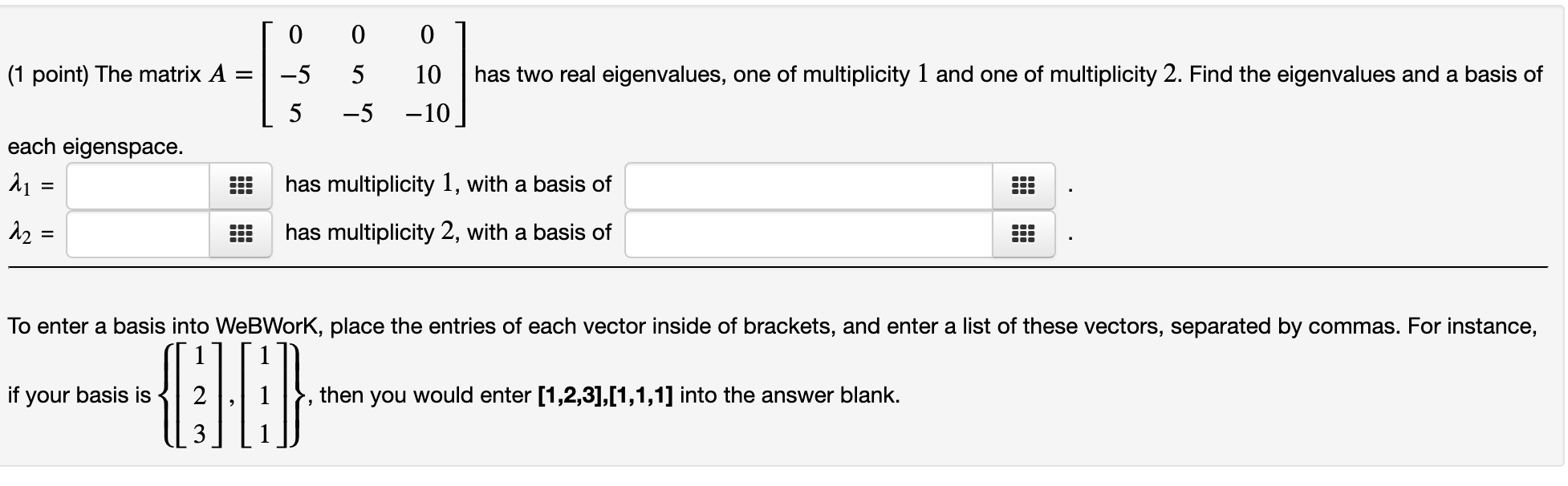T0 0 0 ] (1 point) The matrix A = -5 5 10 has two real eigenvalues, one of multiplicity 1 and one of multiplicity 2. Find the eigenvalues and a basis of [ 5 -5 -10] each eigenspace. 11 = has multiplicity 1, with a basis of 22 = !! has multiplicity 2, with a basis of 010 To enter a basis into WebWork, place the entries of each vector inside of brackets, and enter a list of these...

• ### (1 point) Let A-0 -2 3 Find a basis of nullspace(A). Answer: To enter a basis...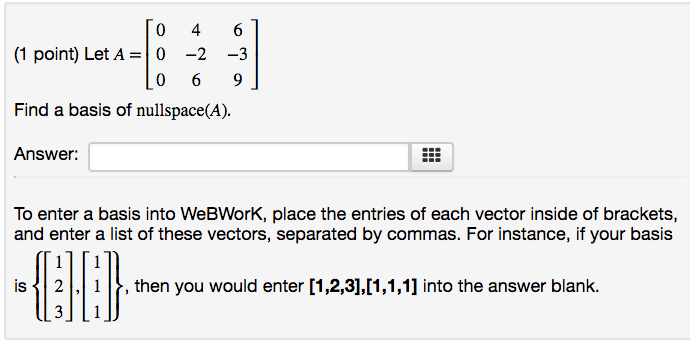(1 point) Let A-0 -2 3 Find a basis of nullspace(A). Answer: To enter a basis into WeBWorK, place the entries of each vector inside of brackets, and enter a list of these vectors, separated by commas. For instance, if your basis is 21 , then you would enter [1,2,3],11,1,1] into the answer blank.

• ### (1 point) Let L be the linear operator in R? defined by L(x) = (4x1 –...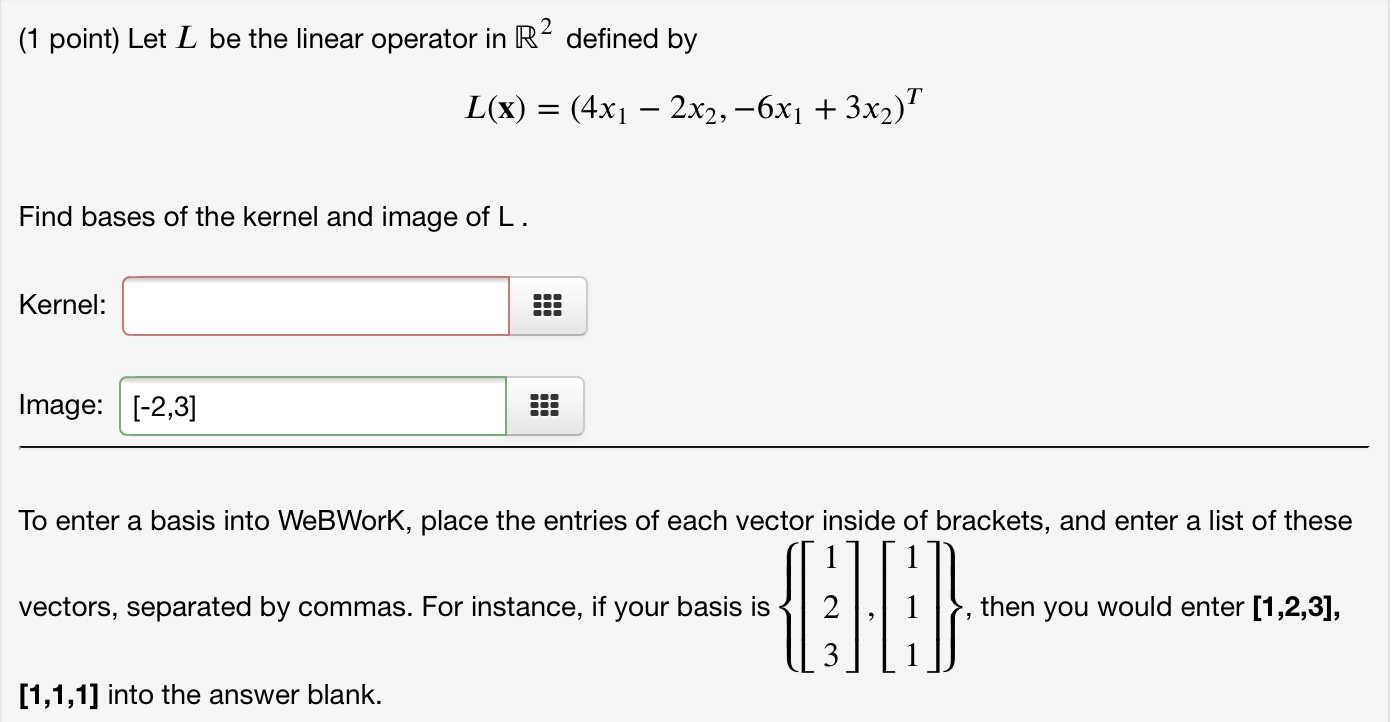(1 point) Let L be the linear operator in R? defined by L(x) = (4x1 – 2x2, -6x1 + 3x2) Find bases of the kernel and image of L. 00 Kernel: * Image: [-2,3] To enter a basis into WebWork, place the entries of each vector inside of brackets, and enter a list of these vectors, separated by commas. For instance, if your basis is 1 2,1l/, then you would enter [1,2,3], 31 [1,1,1) into the answer blank.

• ### Section 3.4 Basis and Dimension: Problem 4 Previous Problem Problem List Next Problem (1 point) Find...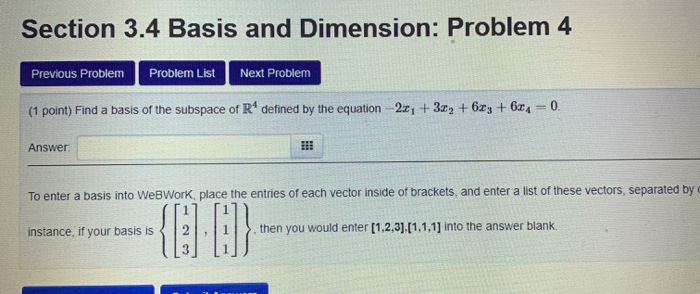Section 3.4 Basis and Dimension: Problem 4 Previous Problem Problem List Next Problem (1 point) Find a basis of the subspace of R* defined by the equation - 2:04 +32 +673 +624 = 0 Answer To enter a basis into WebWork, place the entries of each vector inside of brackets and enter a list of these vectors, separated by instance, if your basis is 2 . 1 , then you would enter [1,2,3],[1,1,1) into the answer blank.

• ### please give the correct answer with explanations, thank you Als a 3 x 4 matrix with...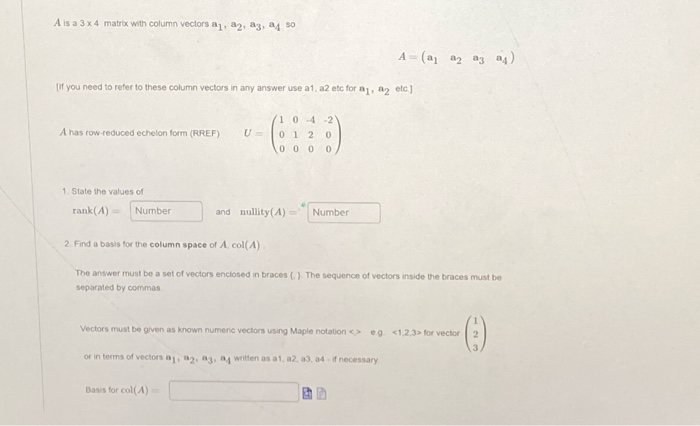please give the correct answer with explanations, thank you Als a 3 x 4 matrix with column vectors a, a, a3, 24 50 A (a a2 a3 24 If you need to refer to these column vectors in any answer use a1 a2 etc for 1, 2 etc.) A has row reduced echelon form (RREF) 1042 0 1 2 0 0 0 0 1. State the values of rank(A) Number and nullity(A) Number 2. Find a basis for the column...

• ### Find a basis of the subspace of R4 that consists of all vectors perpendicular to both...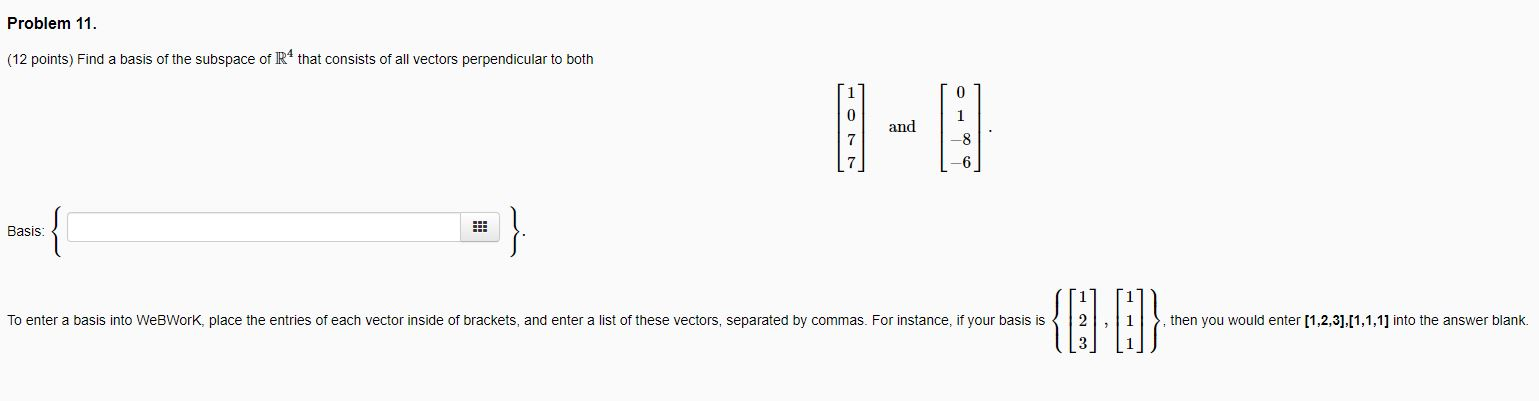Find a basis of the subspace of R4 that consists of all vectors perpendicular to both Problem 11. (12 points) Find a basis of the subspace of R4 that consists of all vectors perpendicular to both Basis: 111 To enter a basis into WebWork, place the entries of each vector inside of brackets, and enter a list of these vectors, separated by commas. For instance, if your basis is was to me, you are » {]J (1) mar yavros en...

• ### Given the matrix A and its reduced row echelon form R, answer the following questions. 4...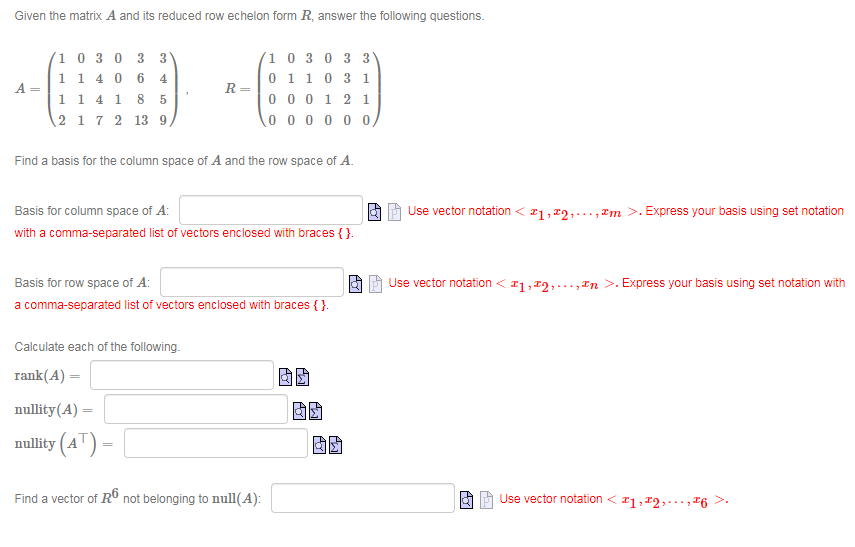Given the matrix A and its reduced row echelon form R, answer the following questions. 4 A 1 0 3 0 3 3 1 1406 1 1 4 1 8 2 1 7 2 13 9 R= 1 0 3 0 3 3 0110 31 000121 1000000 5 Find a basis for the column space of A and the row space of A. Ao Basis for column space of A: with a comma-separated list of vectors enclosed with braces {}....

• ### Please answer the following. Thank you. (1 point) Let A--5-5-5 5 |. Find basis for the...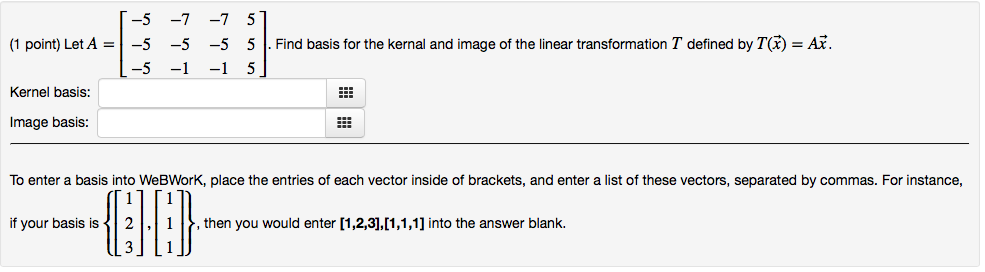Please answer the following. Thank you. (1 point) Let A--5-5-5 5 |. Find basis for the kernal and image of the linear transformation T defined by T(刃 L-5-1 5, Kernel basis: Image basis: To enter a basis into WeBWorK, place the entries of each vector inside of brackets, and enter a list of these vectors, separated by commas. For instance, if your basis is2 1 I&, then you would enter [1,2,3],[1,1,1] into the answer blank. 3] L1 (1 point) Let...